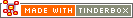sum_if(group,condition,value)Operator Type: Boolean Operator Scope of Action: Conditional Group

The operator sum_if() adds lists. (See sum() for a related new operator)

sum_if(where,condition,what)

visits each note described by where, testing if it meets the condition and adds the value of the designated attribute to the total.

where may be any of {child,descendent,sibling,ancestor,all}.

In addition, where may be argument that designates a particular (single) note other than this.

condition is a valid conditional test - i.e. it equates to true when matched.

what can be any expression, but is typically an attribute. It can also be the number 1, i.e. if the test is true from that item then add one to the count.

For example,

`sum_if(child,\$Prototype=="p_Problem",1)`

sums the number of children of the current note whose prototype is 'p_Prototype'. If tested value is a string with spaces, e.g. "p Prototype" vs. "p_Prototype" then use double quotes around the value.

 Up: Conditional Group-based operators Previous: collect_if(group,condition,attribute)

[Last updated: 14 Dec 2009, using v5.0]